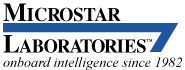Building PID Controls in Software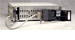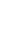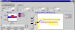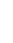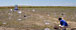Practical PID Controls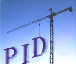(View more Software Techniques or Control Applications and Techniques.)

The DAPL system has supported PID controls since its very earliest versions. The reason for doing this was to enable developers to use exactly the same methods that the pre-defined PID command used, for building customized controller commands. The reason that this maybe wasn't such a good idea, it limited developers to using exactly the same methods!

Actually, a system interface with multiple service functions is not necessary to support PID. As you will see, practical PID controls only require about 20 lines of run-time code. You can put in the same 20 or so lines into your processing command and you will have all of the same capabilities, plus easy access for modifying the controller to meet special needs.

PID state

Your PID controller needs two kinds of data: configuration and state. The configuration contains the adjustable settings that persist over time.

class  PID_params
{
// Gain parameters
public:
float  Kgain;        // Loop gain parameter
float  Ti;           // Integrator time constant
float  Td;           // Differentiator time constant
float  delT;         // Update time interval

// Setpoint parameters
float  setpt;        // Regulated level to maintain
} ;

The internal state variables change from sample to sample depending on what happens in the feedback.

class  PID_state
{
// Controller state
public:
float  integral;   // Summation of setpoint errors
float  deriv;      // Previous setpoint error
} ;

The main reason for organizing the data in this manner is convenient addressing. State and gain settings are used together all the time at runtime, so it is not a terrible violation of encapsulation principles to merge the structures into one common PID_data object.

PID Initializations

Before the PID computations begin, set up the PID parameter and state objects, and initialize all of the terms.

PID_params   Params;
PID_state    State;
// Initialize these...

PID computations will implement the following control law.

u  =  -Kp * ( err + integral(err)/Ti + deriv(err)*Td )

When approximating the integral using rectangular rule integration, the integral adjustment at each time step becomes the integrand (setpoint error) times the update time interval. When approximating the derivative using a first difference, the deriviative estimate is the difference in successive setpoint error values divided by the update time interval.

PID Computations

The PID computations compare the actual system output to the setpoint to determine the setpoint tracking error. This difference drives the proportional correction.

seterr = curr_feedback - PID_params.setpt;
// Proportional response
pidout = seterr;

The setpoint error drives the integrator, which then drives the PID response indirectly. After the integral value is used, its state is updated for the next pass.

pidout += PID_state.integral * PID_params.delT / PID_params.Ti;
PID_state.integral += seterr;

The derivative of the setpoint error is needed next. The actual derivative is seldom available, so the PID controller estimates the derivative value using a difference approximation. This calculation requires keeping one previous value in state memory.

change = seterr - PID_state.deriv;
pidout += change * PID_params.Td / PID_params.delT;
PID_state.deriv = seterr;

Finally, the control output is generated by applying the control gain.

pidout *= -PID_state.Kgain;
// drive controller output

When somebody says they are using PID control, this is the complete story. So far, only 8 lines of run-time code are used to implement it. As a practical matter, you will probably want a few common modifications, and these are covered next.

Coping with Limits

Most software-driven PID controllers will need to convert the computed gains into a fixed-point value and use this to drive a digital to analog output converter. There is no practical bound on the value that the PID control could compute, but there is a definite limit on the fixed point number range to which output converters can respond correctly. At a minimum, it is prudent to limit the output to that range.

There can be good reasons for more restrictive limits: amplifiers that are unipolar and can't respond to negative values, systems that respond dangerously fast if driven too hard, etc. We can add two additional configuration parameters and force outputs to be limited to the specified range.

// New variables for PID_params
float lowlim;
float highlim;
...

// Enforce output limits
if  (pidout > PID_params.highlim)
pidout = PID_params.highlim;
if  (pidout < PID_params.lowlim)
pidout = PID_params.lowlim;
// drive controller output

Avoiding Windup

After limits are applied, linear PID controls become nonlinear, and this has some side effects. Consider what happens when a controller starts at a zero state and is commanded to start regulating at a high level. While the controller is driving hard toward the new level, the system is still far away from the target setpoint, so the integral accumulates rapidly. Eventually, even though the desired output level is reached, and passed, the integral effects by themselves are enough to continue driving ahead at maximum. This behavior is known as windup, and the extended transient time required to correct the integrator imbalance is known as unwinding. Various strategies to counter windup effects are known collectively as anti-windup.

1. Integrator Latching. Since accumulation problems occur predominantly while the control output is at a limit, do not allow integrator adjustments during this time. Defer updating the integrator state until after limits are checked.
// Enforce output limits and anti-windup latch
if (pidout >= PID_params.highlim)
pidout = PID_params.highlim;
else if (pidout <= PID_params.lowlim)
pidout = PID_params.lowlim;
else
PID_state.integral += seterr;
// drive controller output
1. Soft Integrator Anti-Windup. As it cures the windup problem, the integral clamping strategy sometimes delays desirable integrator action. The soft anti-windup strategy reduces integrator changes rather than completely eliminating them. Select a reduction factor in the range 0.05 to 0.25.
// New variable for PID_params
float  anti_windup;
...

// Enforce output limits and soft anti-windup
if (pidout >= PID_params.highlim)
{
pidout = PID_params.highlim;
PID_state.integral += anti_windup * seterr;
}
else if (pidout <= PID_params.lowlim)
{
pidout = PID_params.lowlim;
PID_state.integral += anti_windup * seterr;
}
else
PID_state.integral += seterr;
// drive controller output ...

A reduction factor of 0 is the same as the clamping anti-windup strategy. A reduction factor of 1 is the same as no anti-windup correction.

1. Integrator Rate Limiting. Presuming that the error integral is intended for final settling, not for rapid response to large transients, limit the integrand. This limits how fast the integral can change. An additional configuration parameter is required for the independent integrator rate limiter.
// New variables for PID_params
float  rate_limit;
...

ichange = seterr;
if  (ichange >  PID_params.rate_limit)
ichange =  PID_params.rate_limit;
else if  (ichange < -PID_params.rate_limit)
ichange = -PID_params.rate_limit;
pidout += PID_state.integral * PID_params.delT / PID_params.Ti;
PID_state.integral += ichange;

Removing Command Glitches from Derivative Response

For computing the derivative estimate, the current and previous setpoint errors are subtracted. When regulating a constant setpoint, this difference is exactly the same as subtracting the current and previous feedback values. When the setpoint is changed, however, the change in the setpoint looks like an instantaneous, "near-infinite" spike that hits the derivative gain hard.

For applications where the command level changes continuously and smoothly, the basic derivative scheme works fine. For regulation applications, it is usually better to avoid the setpoint level spikes and use the differences between current and previous feedback explicitly, all of the time.

change = curr_feedback - PID_state.deriv;
pidout += change * PID_params.Td / PID_params.delT;
PID_state.deriv = curr_feedback;

Improving Derivative Response

Derivative control action should oppose rapid changes and should therefore be beneficial — but it has a bad reputation for being "destabilizing."

• It introduces a closed loop zero. Closed loop poles, not zeroes, cause instability. Still, the "peaking" gains produced at high frequencies can exaggerate oscillations and intefere with gain margins.
• The higher the frequency, the less likely the relevance to the control problem, but the more the derivative term amplifies it. The derivative term tends to increase noise.
• The derivative term is an estimate, and errors in the estimate can in themselves be a source of noise.
• The derivative feedback does not play well with time delays. A derivative gain that is fine in a continuous variable controller might be destabilizing in combination with the time delay of a discrete-time control loop.

The problems are worst at the Nyquist frequency, with a tendency to produce a high-to-low rattling that damps slowly.

A derivative frequency response increases monotonically at high frequencies. A lowpass filter can offset this gain and neutralize phase shifts at higher frequencies. The lowpass filter needs to have minimal effect at the important lower frequencies, while providing attentuation of high frequencies.

Two filtering strategies can help with this.

1. Averaging filter. This introduces a transmission zero at the Nyquist frequency. One additional state variable is needed.
// New variable for PID_state
float   oldderiv;
...

change = (seterr - PID_state.oldderiv)/2;
pidout += change * PID_params.Td / PID_params.delT;
PID_state.oldderiv = PID_state.deriv;
PID_state.deriv = seterr;
1. Single pole filter. This requires an additional lag variable for filter state, plus an additional parameter between 0.0 and 1.0 to configure the cutoff frequency.

This scheme corresponds to the lag filtering usually assumed in analog simulations.
// New variable for PID_params
float   lagcut;
...

// New variable for PID_state
float   lagstate;
...

change = seterr - PID_state.deriv;
PID_state.lagstate = (1.0-PID_params.lagcut)*PID_state.lagstate
+ (PID_params.lagcut)*change;
pidout += PID_state.lagstate * PID_params.Td / PID_params.delT;

A lag parameter value of 0.15 to 0.35 usually works well. The lower this cutoff level, the better the high frequency noise rejection but the more likely that effectiveness of the derivative term is reduced.

Gain changes that are applied automatically and continuously, as in the case of an adaptive tuning scheme, are small and introduced so slowly that there is no visible impact. But gain changes that are larger can produce an artificial transient. This is an avoidable problem.

No special adjustments are required for the derivative or proportional feedback effects. In steady operation the derivative term does not respond. When the output is at the regulated level, the setpoint error is very small and so is the effect of proportional response. During transients the effects of a gain adjustment would not be noticed.

That leaves the integral term. The loop gain and integral time constant terms act together upon the current integral value to hold the loop output at the regulated level. If you change either of these two gain terms, there will be an instantaneous level change in the control output. Ordinarily, what you will want instead is that the output level remains as it was before applying gain adjustments.

The effect of the integral term before gain adjustments is

integralold * Kpold/Tiold

To avoid an artificial transient, you must artificially adjust the integral value at the same time as the gains, so that the net effect of the integral term remains the same.

integralnew = integralold * (Kpold/Kpnew) / (Tiold/Tinew)

After this adjustment, the new values of loop gain Kgain and integral time constant Ti can replace the previous values in the PID_params object.

Unbalanced Output Drive

It is not uncommon to find that the control loop works against a biased loading. For example, an actuator applies lift, and must work against gravity to move its load upward, but it must work with gravity to move the load downward. Ordinarily, PID action is the same in both directions, and this leads to pulling downward too hard while not pushing upward hard enough.

The proportional term is intended for responding quickly to deviations from the setpoint. The amount of adjustment to apply is indicated by an additional parameter. The sign of the setpoint tracking error can be tested to determine whether to increase or decrease proportional response according to the new parameter.

// New variable for PID_params
float  delKp;
...

seterr = curr_feedback - PID_params.setpt;
if  (seterr >= 0.0)
seterr *= delKp;
else
seterr /= delKp;

// Proportional response
pidout = seterr;

Feedforward Compensation

Sometimes it is necessary to control a system that has a tendency to "ring." The oscillations damp out slowly, and there is not much that can be done, but PID controls should avoid causing them. Abrupt level changes can contribute energy at the frequency of ringing and excite the oscillations unnecessarily.

There are two techniques that you can apply.

1. Adjustable command path gain. The proportional action is split between the feedback response and the setpoint command response, and different gains are applied on the two paths. The effect is to shift the transfer function zero that the PID controller introduces, moving it away from the frequency where the oscillation occurs, reducing interactions that might encourage the oscillation. This option requires an additional gain scaling parameter that must be tuned along with the usual PID gains. The Kz parameter takes a value from 0.0 to 1.0. At 1.0, the loop action is the same as classic PID.
// New variable for PID_params
float   Kz;
...

// No change for integral and derivative terms
seterr = curr_feedback - PID_params.setpt;
// Proportional response differs for command and feedback
pidout = curr_feedback - Kz * PID_params.setpt;
1. Pre-filtering. Smoothing out sharp edges in the command signal avoids frequencies that might excite an oscillation. The filtering causes delay, but because this occurs on the command signal before driving the loop, not in the feedback path, this delay has no impact on stability.

The design of the filter is beyond the scope of this paper. The smoothed setpoint signal with gradual level changes is then applied to drive the PID command instead of using the setpoint changes directly. This could be a separate filtering task, but the filter and the PID gains often are closely related and sometimes more easily managed in one location.

If the filter reduces to a simple gain less than 1, this becomes the same as the adjustable command gain strategy.

Conclusions

This note has described how PID software controls work, and how to implement them in custom processing commands. If there is anything complicated, you didn't see it here. Strictly speaking, PID controls are linear controls with three gain parameters, but most implementations will apply one or more of the extensions. There are many more possibilities, but, beyond a certain point, it is questionable whether the exotic variants deserve being called PID control.

The variants are shown here in an informal style that should be comfortable both to C and C++ programmers.

While we have covered the 20 or so lines of programming code needed for the PID computations, the real challenges will lie in getting data into the computations, and getting results out of the computations, in a manner that will meet real-time requirements. Additional support in the form of fully functional command examples is provided in the Developer's Toolkit for DAPL.

(View more Software Techniques or Control Applications and Techniques.)